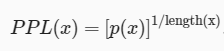# [Information Theory] Potential issue with part 3 of 3rd exercise in the section 18.11.7

Hi everyone,

I am trying to solve the problems given in the Information Theory section. I am stuck at part 3 of 3rd exercise. It mentions that the perplexity of every character is 15 points but the formula given in the problem isn’t backing this number.

The formula isI have two doubts here -

1. The definition of perplexity given in the problem isn’t the one given on Wikipedia. Could you please explain what do the two x in the equation mean?
2. Assuming this definition to be correct, 15 doesn’t seem to be a possible value because both `p(x)` and `1\length(x)` have values less than or equal to 1. So, the upper bound of `p(x)^(1\length(x))` is 1.

Could you please let me know what am I missing here? Thanks

Sorry. I can’t reply you. @goldpiggy is the author. Maybe she can.

Maybe we need to open more new topics for remaining chapters ASAP to avoid these type to happen.

Please let me know if it is clear to you?!